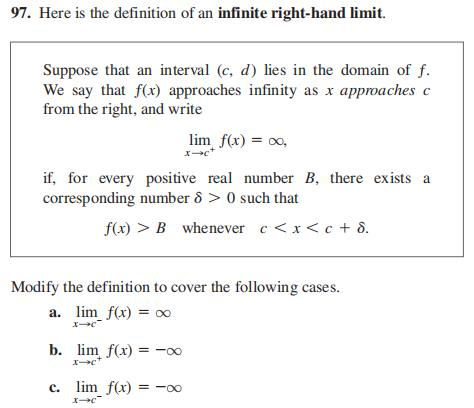### Still have math questions?

Trigonometry
Question97. Here is the definition of an infinite right-hand limit. Suppose that an interval $$( c , d )$$ lies in the domain of $$f$$ . We say that $$f ( x )$$ approaches infinity as $$x$$ approaches $$c$$ from the right, and write $$\lim _ { x \rightarrow c ^ { + } } f ( x ) = \infty$$ , $$f ( x ) > B$$ whenever $$c < x < c + \delta$$ if, for every positive real number $$B$$ , there exists a corresponding number $$\delta > 0$$ such that Modify the definition to cover the following cases.

a. $$\lim _ { x \rightarrow c ^ { - } } f ( x ) = \infty$$

b. $$\lim _ { x \rightarrow c ^ { + } } f ( x ) = - \infty$$

c. $$\lim _ { x \rightarrow c ^ { - } } f ( x ) = - \infty$$

$$\left. \begin{array} { l } { \text { (a) For every positive real number } B \text { there exists a corresponding } } \\ { \text { number } \delta > 0 \text { such that for all } x } \\ { \qquad c - \delta < x < c \Rightarrow f ( x ) > B } \end{array} \right.$$